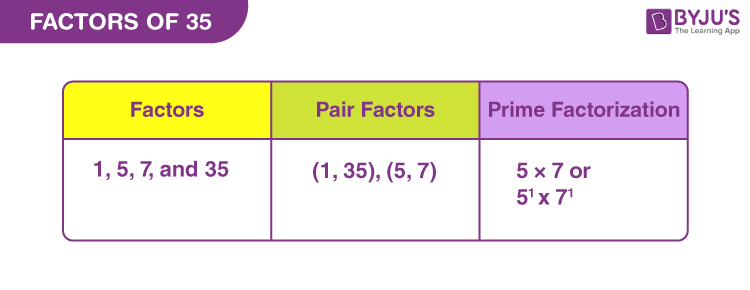# Factors Of 35

The factors of 35 are the numbers that divide 35 exactly without leaving a remainder value. There is a total of four factors for 35, in which 35 is the largest factor and 1 is the smallest factor. These factors are 1, 5, 7 and 35. If two numbers are multiplied in pairs resulting in the original number, then it is called the pair factor of 35. These pair factors are (1, 35) and (5, 7). Hence, these factors are the positive integers but cannot be a decimal or fraction.  If we add all the factors of 35, then the sum will be equal to 48.

## What are the Factors of 35?

In Mathematics, the numbers that divide 35 exactly are the factors of 35. It means a  number should divide 35 completely and leave the remainder 0. As 35 is a composite number, it has more than two factors. Thus, the factors of 35 are 1, 5, 7 and 35. Similarly, the negative factors of 35 are -1, -5, -7 and -35.## Pair Factors of 35

If two numbers are multiplied together to get the number 35, then the pair of numbers is called the pair factor of 35. As discussed above, we have both positive and negative pair factors of 35. The positive and negative pair factors of 35 are given below:

Positive Pair Factors of 35:

 Positive Factors of 35 Positive Pair Factors of 35 1 × 35 (1, 35) 5 × 7 (5, 7)

Negative Pair Factors of 35:

 Negative Factors of 35 Negative Pair Factors of 35 -1 × -35 (-1, -35) -5 × -7 (-5, -7)

## How to Find Factors of 35?

There are two methods by which we can find the factors of 35, they are:

• Division method
• Prime factorisation method

## Factors of 35 by Division Method

In the division method, the factors of 35 can be found by dividing 35 by different integers. If the integer divides 35 completely and leaves the remainder 0, then the integers are the factors of 35. Now, let us start dividing 35 by 1 and continue with different integers.

• 35/1 = 35 (Factor is 1 and Remainder is 0)
• 35/5 = 7 (Factor is 5 and Remainder is 0)
• 35/7 = 5 (Factor is 7 and Remainder is 0)
• 35/35 = 1 (Factor is 35 and Remainder is 0)

If we divide 35 by any numbers other than 1, 5, 7 and 35, it leaves a remainder of some value. Hence, the factors of 35 are 1, 5, 7 and 35.

## Prime Factorization of 35

The process of writing the number 35 as the product of its prime factors is called the prime factorization of 35. Now, let us discuss how to find the prime factors of 35 using the prime factorization method.

• Take the pair factor of 35, say (1, 35)
• We know that the number 1 cannot be factored further. So, take the other number 35, which is a composite number
• Now, split the composite number into its prime factors
• Thus, the number 35 can be written as the product of 5 and 7.
• Now, both the numbers 5 and 7 are the prime factors. Hence, 35 = 5 × 7

Therefore, the prime factorization of 35 is 5 × 7 or 51 × 71.

## Video Lesson on Prime Factors### Factor Tree of 35### Facts to be Noted

• Factors of 35: 1, 5, 7 and 35
• Pair Factors of 35: (1, 35) and (5, 7)
• Prime factors of 35: 5 and 7
• Prime factorisation of 35: 51 x 71

## Related Articles

 Links Related to Factors Factors of 15 Factor of 36 Factors of 48 Factors of 18 Factors of 42 Factors of 60 Factors And Multiples

## Solved Examples on Factors of 35

Example 1:

Find the common factors of 35 and 34.

Solution:

The factors of 35 are 1, 5, 7 and 35.

The factors of 34 are 1, 2, 17 and 34.

Hence, the common factor of 35 and 34 is 1.

Example 2:

Find the common factors of 35 and 36.

Solution:

Factors of 35 = 1, 5, 7 and 35.

Factors of 36 = 1, 2, 3, 4, 6, 9, 12, 18, and 36.

Hence, the common factor of 35 and 36 is 1.

Example 3:

Find the common factors of 35 and 17.

Solution:

The factors of 35 are 1, 5, 7 and 35.

The factors of 17 are 1 and 17.

As 17 is a prime number, the common factor of 35 and 17 is 1 only.

## Frequently Asked Questions on Factors of 35

Q1

### What are the factors of 35?

The factors of 35 are 1, 5, 7 and 35.

Q2

### What is the prime factorization of 35?

The prime factorization of 35 is 5 × 7.

Q3

### What are the positive pair factors of 35?

The positive pair factors of 35 are (1, 35) and (5, 7).

Q4

### Mention the negative pair factors of 35.

The negative pair factors of 35 are (-1, -35) and (-5, -7).

Q5

### Is 7 a factor of 35?

Yes, 7 is a factor of 35. If 35 is divisible by 7, it leaves a remainder 0, and hence 7 is a factor of 35.

Test your knowledge on Factors Of 35